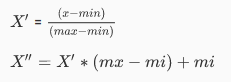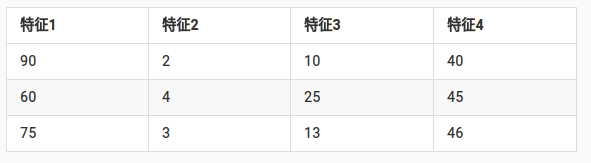2018/04/14 - 次.

# Scik-Learn之特征工程(二)

## 数据的特征预处理

sklearn特征处理API：sklearn.preprocessing

• 数值型数据：标准缩放：

• 1、归一化

• 2、标准化
• 3、缺失值
• 别型数据：one-hot编码
• 时间类型：时间的切分

#### 归一化sklearn归一化API

``````sklearn.preprocessing.MinMaxScaler
``````
###### MinMaxScaler语法
• MinMaxScalar(feature_range=(0,1)…)
• 每个特征缩放到给定范围（默认[0,1])
• MinMaxScalar.fit_transform(X)
• X:numpy array格式的数据[n_samples,n_features]
• 返回值：转换后的形状相同的array
###### 案例测试
``````from sklearn.preprocessing import MinMaxScaler

mydata = [[90,2,10,40],
[60,4,15,45],
[75,3,13,46]]

# 创建归一化实例对象
unificati = MinMaxScaler()

# 转换为归一化特征数据
mydatas = unificati.fit_transform(mydata)

print('归一化特征数据：\n',mydatas)
``````

`````` [[1.         0.         0.         0.        ]
[0.         1.         1.         0.83333333]
[0.5        0.5        0.6        1.        ]]
``````